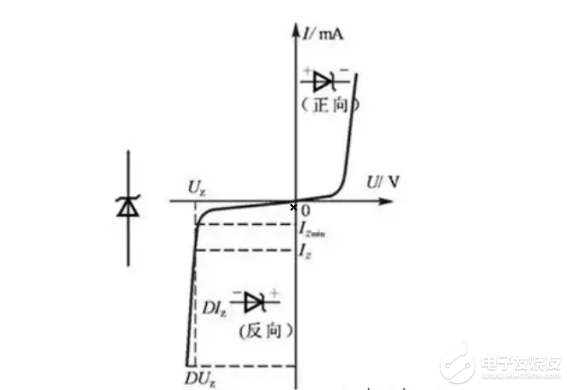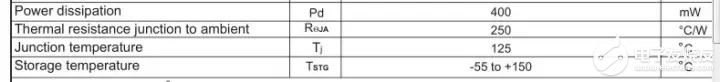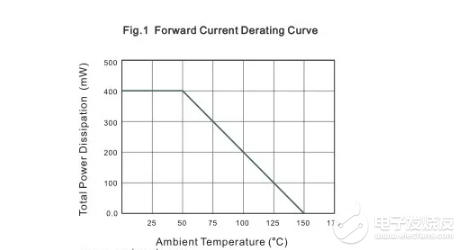How to avoid diode overload damage?
• 所属分类：【Product news】
• 发布日期：2022-03-26 10:38:11
• 浏览次数：
Dissipative power, also known as collector maximum allowable dissipative power PCM, refers to the maximum collector dissipative power when the change of transistor parameters does not exceed the specified allowable value It refers to the active power tra
Dissipative power, also known as collector maximum allowable dissipative power PCM, refers to the maximum collector dissipative power when the change of transistor parameters does not exceed the specified allowable value. Is the difference between the total power of active input and the total power of active output of power grid elements or the whole network at a certain time. Under the linear condition, the calculation of conduction dissipation power is relatively simple, PD = I2R, or PD = U2 / R; In the on-off state, the calculation is relatively complex.

The dissipation power of diode is related to the allowable temperature saving. The maximum allowable temperature saving of silicon diode is 150 ℃, while that of germanium is 85 ℃. The working temperature of semiconductor is limited. When the actual power increases, its temperature saving will also increase. When the temperature saving reaches 150 ℃, the power at this time is the maximum dissipated power. Of course, the dissipative power also has a certain relationship with the package size. Generally, the maximum dissipative power of devices with larger packages is also relatively large. The most common is that high-power devices have large volume and large area of heat dissipation metal surface.The dissipated power of a specific type of diode is related to the test conditions, such as the test ambient temperature and heat dissipation conditions. Usually, the maximum dissipation power tested is at 25 ℃. With the increase of ambient temperature, the maximum dissipation power will decrease, because the heat conduction temperature difference under this condition becomes smaller. For example, at 25 ℃, the dissipation power of a diode can reach 1W, and at 75 ℃, the dissipation power may become 0.4W. The maximum allowable dissipation power is related to the heat dissipation conditions. The better the heat dissipation conditions, the higher the dissipation power. At the same ambient temperature, the dissipation power is 1W. After adding heat sink, the dissipation power may become 1.7w.

One parameter characterizing heat dissipation measures is thermal resistance. Thermal resistance is a comprehensive parameter that reflects the ability to prevent heat transfer. Thermal resistance is similar to resistance in electronics. It is a reference quantity reflecting the "blocking ability". The smaller the thermal resistance, the stronger the heat transfer capacity; On the contrary, the greater the thermal resistance, the smaller the heat transfer capacity. From the perspective of analogy, heat is equivalent to current, temperature difference is equivalent to voltage, and thermal resistance is equivalent to resistance. Among them, thermal resistance RJA: the total thermal resistance of the heat source of the chip to the surrounding cooling air, and its unit is ℃ / W, which represents the temperature difference between the two ends of heat conduction under 1W.

Taking MDD 1n4448ws as an example, by checking its specification, it can be seen that its thermal characteristics are as follows:It can be seen that the dissipated power PD = 400MW and the thermal resistance is 250 ℃ / W, of which these two quantities are tested under the condition that the ambient temperature is 25 ℃ and the PCB made of FR-4 is welded. The dissipated power is related to the ambient temperature. The higher the temperature, the smaller the dissipated power. The relationship between 1n4448ws dissipated power and ambient temperature is as follows:At 0 ~ 50 ℃, the dissipated power is constant at 400MW. At 50 ~ 150 ℃, it decreases linearly to 150 ℃, and the dissipated power is 0. At this temperature, the silicon tube can no longer work. From this table, the inverse slope of the linear part of the thermal resistance can be calculated:

|1/k|=Rja=(150-50)/0.4=250℃/W

According to this table, we can get:

PD=-1/250(TA-50)+0.4，(TA-≥50)

According to the above formula, we can calculate the maximum dissipated power of 1N4148WS switch at different ambient temperatures.

In the actual circuit design process, most R & D engineers pay more attention to the working temperature of the device to ensure a safe working range. Taking MDD 1n4448ws as an example, when the ambient temperature is 50 ℃ and the actual power is 200MW, its temperature is 50 + 250 * 0.2 = 100 ℃, and it can work normally; When the actual power is 400MW, the temperature is 50 + 250 * 0.4 = 150 ℃. At this time, the maximum temperature of temperature saving has been reached, which is dangerous and should be avoided.

In terms of heat transfer of diodes, PD and thermal resistance RJA are mainly considered. The former is the maximum dissipation power, which can not be exceeded in actual work, and the latter is the heat transfer resistance parameter, which reflects the heat transfer capacity of different diodes. When using diodes, not only the forward current, reverse withstand voltage and switching time, but also the dissipated power should be considered.

The scientific use of diodes makes the circuit more intelligent. The professional specifications are much stricter than the actual needs.